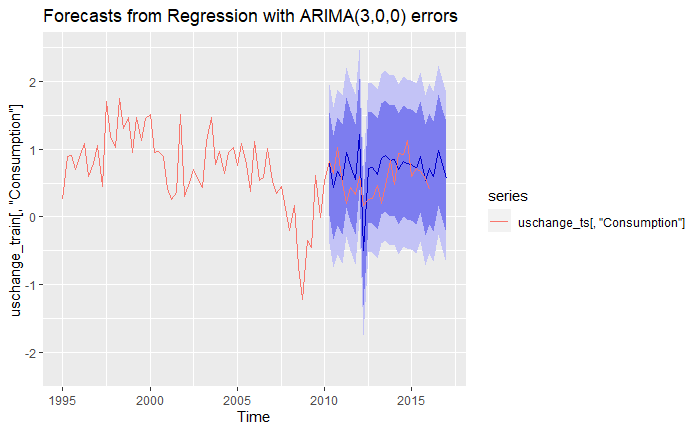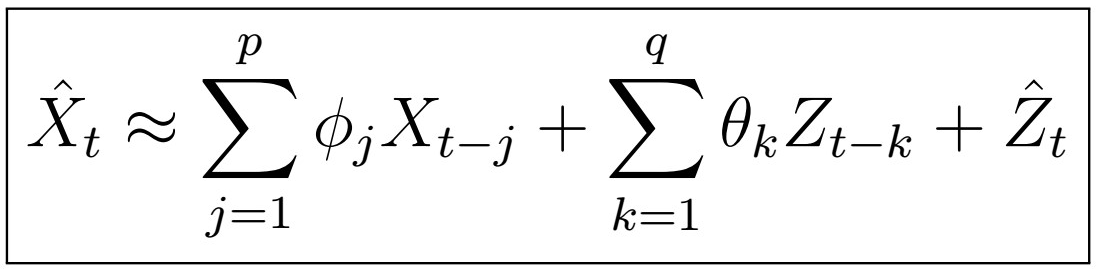# A Complete Introduction To Time Series Analysis (with R):: Exogenous models# A Complete Introduction To Time Series Analysis (with R):: Model Selection for ARMA(p,q)

## Maximum Likelihood Model Selection

In the previous section, we saw how Gaussian assumptions allow us to obtain and maximize the likelihood of some ARMA(p,q) process to obtain parameter estimates for the thetas and alphas. That is, for any model, we are looking to find parameters such that

# A Complete Introduction To Time Series Analysis (with R):: Estimation of ARMA(p,q) Coefficients (Part II)The ARMA(p,q) model implies that X_{t} can be expressed in the form above.

## Estimation of MA(q) (Innovations)

As you may guess by the title, the way to estimate the MA(q) coefficients is… the Innovations Algorithm we saw before. Recall that the MA(q) process can be written as

# A Complete Introduction To Time Series Analysis (with R):: Estimation of ARMA(p,q) Coefficients (Part I)

## Estimation of AR(p) :: Yale-Walker

In real world problems, the ACVF is the easiest thing to estimate using the sample data…

# A Complete Introduction To Time Series Analysis (with R):: Prediction III: Forecasting with ARMA(p,q) models